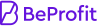###### CompanyPricing
Want to see our dashboard in action?
you can try it here with theDemo Store!

# Gross profit vs. net profit margin for calculating profitability?

If I were calculating the profitability of a business, would it be better for me to use the gross profit margin or the net profit margin? Are they both more or less the same calculation, or is one a more definitive profit margin metric than the other? What are the advantages and disadvantages of using either profit metric for calculating overall profitability?

Izaiah Moss

Saturday, October 16, 2021

﻿Gross profit and net profit are different. A high gross profit margin indicates that a business can make a high profit while the net profit margin shows that a business can make a reasonable profit even after deducting the cost of goods, and other expenses. The gross profit margin is equal to (revenue- the cost of goods sold)/ revenue *100 while the net profit margin is net profit/ revenue *100.

Maurice Mullen

Wednesday, February 09, 2022

People commonly get these terms confused, and often make mistakes when calculating profit margin accurately.

Gross profit margin is the income exceeding the cost of goods sold. It doesn't include the amount paid for taxes or the operational cost of the product. On the contrary, the net profit margin is calculated to be definite about how much profit the company generated. The net profit margin is calculated by subtracting all the expenses, including operational cost, taxes, interest charges, labor cost, etc., from the gross profit. So the net profit tells the actual profit revenue.

You can't calculate the net profit margin without knowing the gross profit margin. Similarly, knowing only the gross profit margin is not enough to measure the organization's profitability.

Curt Cleveland

Sunday, April 24, 2022

Gross profit is an important metric since its necessary when calculating the net profit. The gross profit margin shows that a business can pay the amount required to produce its products. While the net profit subtracts all the business expenses from the revenue. It shows the actual profitability of the businessGross profit is an important metric since its necessary when calculating the net profit. The gross profit margin shows that a business can pay the amount required to produce its products. While the net profit subtracts all the business expenses from the revenue. It shows the actual profitability of the business

Art Barnett

Thursday, September 15, 2022

Gross profit vs. net profit margin: both these terms are entirely different from each other. Gross profit is the overall revenue without including the marketing, interest, and operating expenses. Conversely, the net profit margin is the actual profit the company gained in a specific time after considering all the expenses incurred in the fiscal year.

The above statements show that gross profit is insufficient for a business's perfect profitability measurement. It is pivotal to compute your net profit as well.

To accurately measure the net profit margin, you must calculate the gross profit percentage. The mathematical expression is as follows:

Net Profit = Gross Profit - Total Operating Expenses and Taxes